﻿ 基于乘积季节模型的昆明市月度旅游人数预测

# 基于乘积季节模型的昆明市月度旅游人数预测Forecast of Monthly Tourist Numbers in Kunming Based on the Multiplicative Seasonal Model

Abstract: With the development of our society, tourism industry is playing an increasingly important role. There are all kinds of forecasting models in the studies of travel forecasting. Based on the existing research experience at home and abroad, this article uses the multiple seasonal ARIMA model to fit the monthly tourist population of Kunming from January 2013 to October 2020. Using this model to predict the number of tourists in Kunming in the next two months when the model can pass all the tests.

1. 研究背景及意义

2. 文献综述

3. 模型介绍

3.1. ARMA模型介绍

$\left\{\begin{array}{l}\Phi \left(B\right){\nabla }^{d}{X}_{t}=\Theta \left(B\right){\epsilon }_{t}\\ E\left({\epsilon }_{t}\right)=0,Var\left({\epsilon }_{t}\right)={\epsilon }_{t}^{2},E\left({\epsilon }_{t}{\epsilon }_{s}\right)=0,s\ne t\\ E\left({x}_{t}{\epsilon }_{s}\right)=0,\forall s (1)式

3.2. 季节模型介绍

${x}_{t}={S}_{t}+{T}_{t}+{I}_{t}$ (2)式

${\nabla }_{D}{\nabla }^{d}{x}_{t}=\frac{\Theta \left(B\right)}{\Phi \left(B\right)}{\epsilon }_{t}$ (3)式

${\nabla }^{d}{\nabla }_{s}^{D}{x}_{t}=\frac{\Theta \left(B\right){\Theta }_{s}\left(B\right)}{\Phi \left(B\right){\Phi }_{s}\left(B\right)}{\epsilon }_{t}$ (4)式

$\Theta \left(B\right)=1-{\theta }_{1}B-\cdots -{\theta }_{q}{B}^{q}$

$\Phi \left(B\right)=1-{\varphi }_{1}B-\cdots -{\varphi }_{p}{B}^{p}$

${\Phi }_{s}\left(B\right)=1-{\varphi }_{1}{B}^{s}-\cdots -{\varphi }_{P}{B}^{PS}$

${\Theta }_{s}\left(B\right)=1-{\theta }_{1}{B}^{s}-\cdots -{\theta }_{Q}{B}^{QS}$

3.3. 模型的检验

3.3.1. 模型的显著性检验

$\text{LB}=n\left(n+2\right)\underset{k=1}{\overset{m}{\sum }}\left(\frac{{\rho }_{k}^{2}}{n-k}\right)~{\chi }^{2}\left(m\right),\forall m>0$ (5)式

3.3.2. 参数的显著性检验

3.3.3. 模型选择的依据

4. 数据来源

5. 实证分析

5.1. 原始数据平稳性检验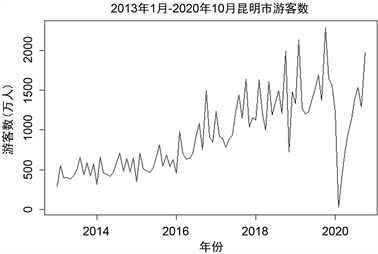Figure 1. Sequence chart of series X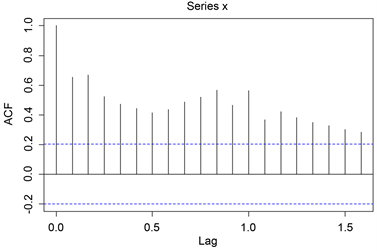Figure 2. Autocorrelogram

5.2. 非平稳数据的处理及检验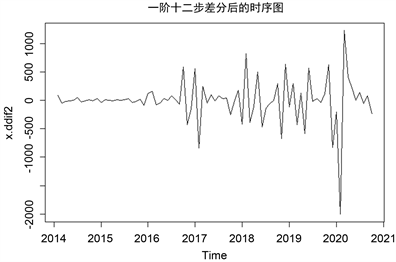Figure 3. Sequence chart of difference seriesTable 1. Unit root stationarity test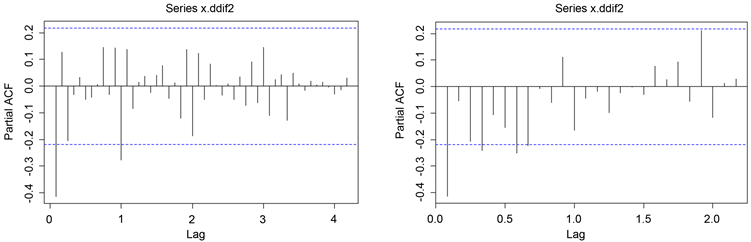Figure 4. Autocorrelation and partial correlation

5.3. 模型的建立与检验

$\text{ARIMA}\left(1,1,1\right)×{\left(0,1,1\right)}_{12}$ 为本文根据数据特征建立的最优月度人数预测模型，其具体形式为：

$\nabla {\nabla }^{12}{x}_{t}=\frac{1-0.8668B}{1-0.3543B}\left(1-0.6885{B}^{12}\right){\epsilon }_{t}$

5.4. 模型的预测Table 4. Comparison between actual and predicted values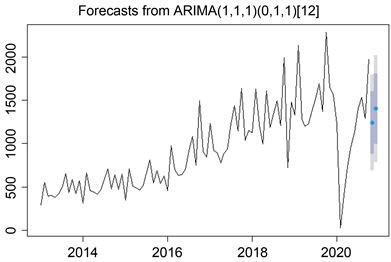Figure 5. Prediction diagram

6. 结论及建议

 陈鹏, 吴玲, 宋徽. 基于ARIMA模型的安徽省入境旅游人数预测[J]. 安徽农业大学学报(社会科学版), 2012, 21(1): 32-35+116.

 孔朝莉. 基于ARIMA模型的海南月度旅游人数预测[J]. 中国科技信息, 2019(5): 76-78.

 刘若愚, 刘立波. 基于ARIMA模型的游客人数分析与预测[J]. 电脑与电信, 2019(Z1): 1-4.

 虞安, 王忠. 基于ARIMA模型与时间序列的城市旅游倾向预测[J]. 统计与决策, 2014(13): 86-89.

 王南丰, 沈李欢. 基于GM(1,1)模型的陕西省旅游人数预测[J]. 甘肃科技纵横, 2020, 49(5): 68-72+67.

 郭况浦, 李信韬. 基于综合预测模型的洛阳市旅游接待需求预测[J]. 内蒙古科技与经济, 2019(1): 27-28+33.

 王政, 杨霞. 云南省入境旅游规模时空分异特征及预测研究[J]. 云南农业大学学报(社会科学), 2020, 14(4): 93-102.

Top